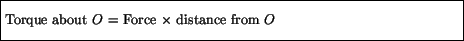Next: Static Equilibrium Up: Rotational Motion Previous: Angular acceleration

# Torque

Again proceeding in analogy with linear motion, we now examine what causes an object to experience rotational motion. Let us consider an object free to rotate about a fixed axis O, as below, and imagine we apply a force F at the indicated point.This force leads to what is called a torque exerted on the object about the point O, defined asTorque is thus measured in units of Newton - meters.

Like force, there is a direction associated with torque, which is related to the net clockwise or counter-clockwise rotation about the pivot point O that the torque causes.

Note that the torque depends on the distance from the point of rotation, and that for a constant force a greater torque results with increasing distance. One can see this by pushing on a door to open it - the further away from the hinges (point of rotation) one pushes, the greater the torque is exerted on the door, and therefore the easier it is to open. In particular, if one pushes on the door directly on the hinges, so that the distance from the point of rotation is zero, no torque results.

In Figure 5.2 the angle between the direction of the applied force and the direct line from the axis O to the point where the force is applied is 90o. If this angle was different from 90o the definition of the torque about O would be modified. In particular, if this angle was zero, so that the force is directed towards the point of rotation, the torque turns out to be zero. Again, one can see this by trying to open a door by pushing on the door towards the hinges; no matter how hard one pushes, the door will not move.Next: Static Equilibrium Up: Rotational Motion Previous: Angular acceleration
modtech@theory.uwinnipeg.ca
1999-09-29